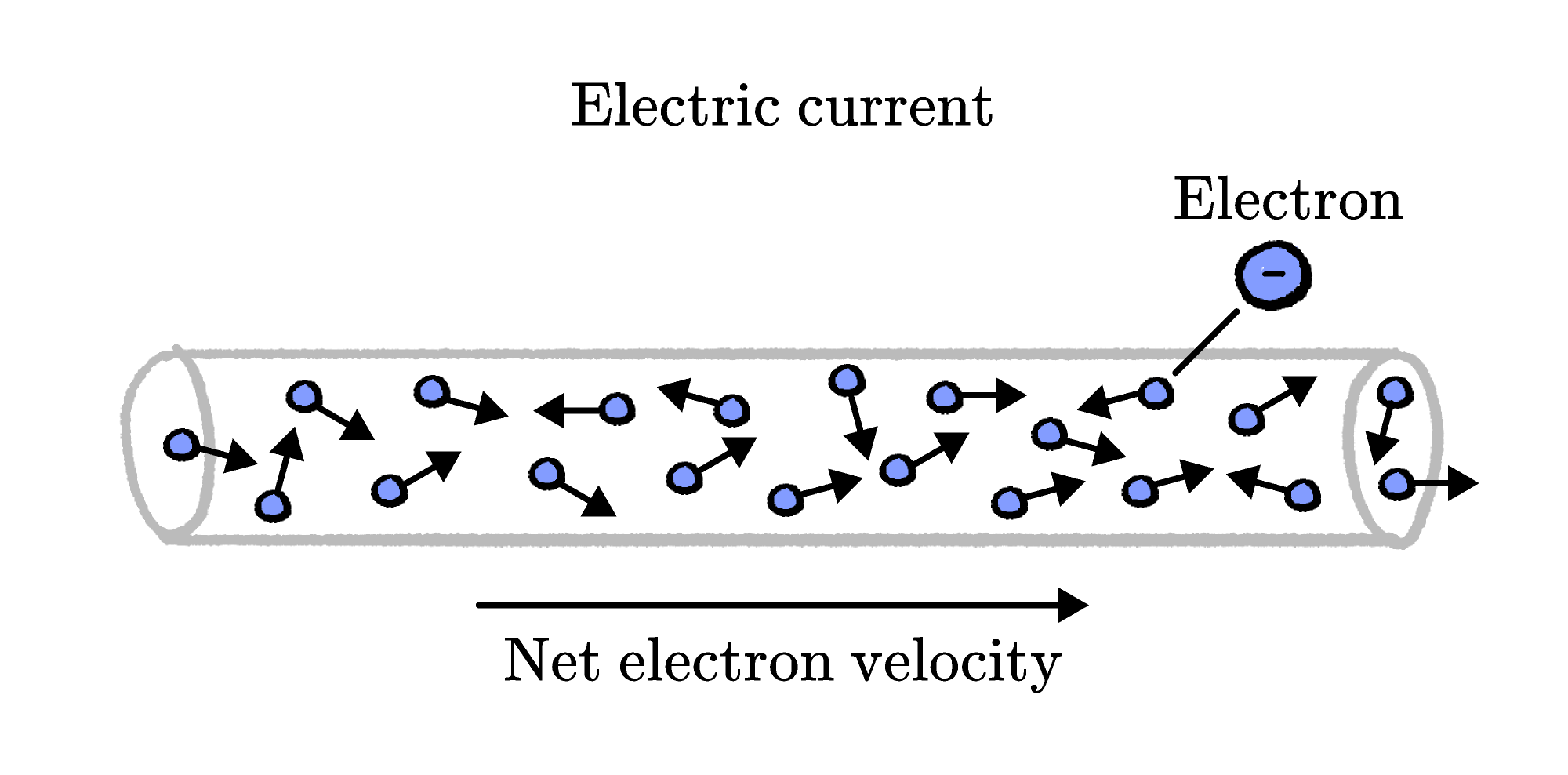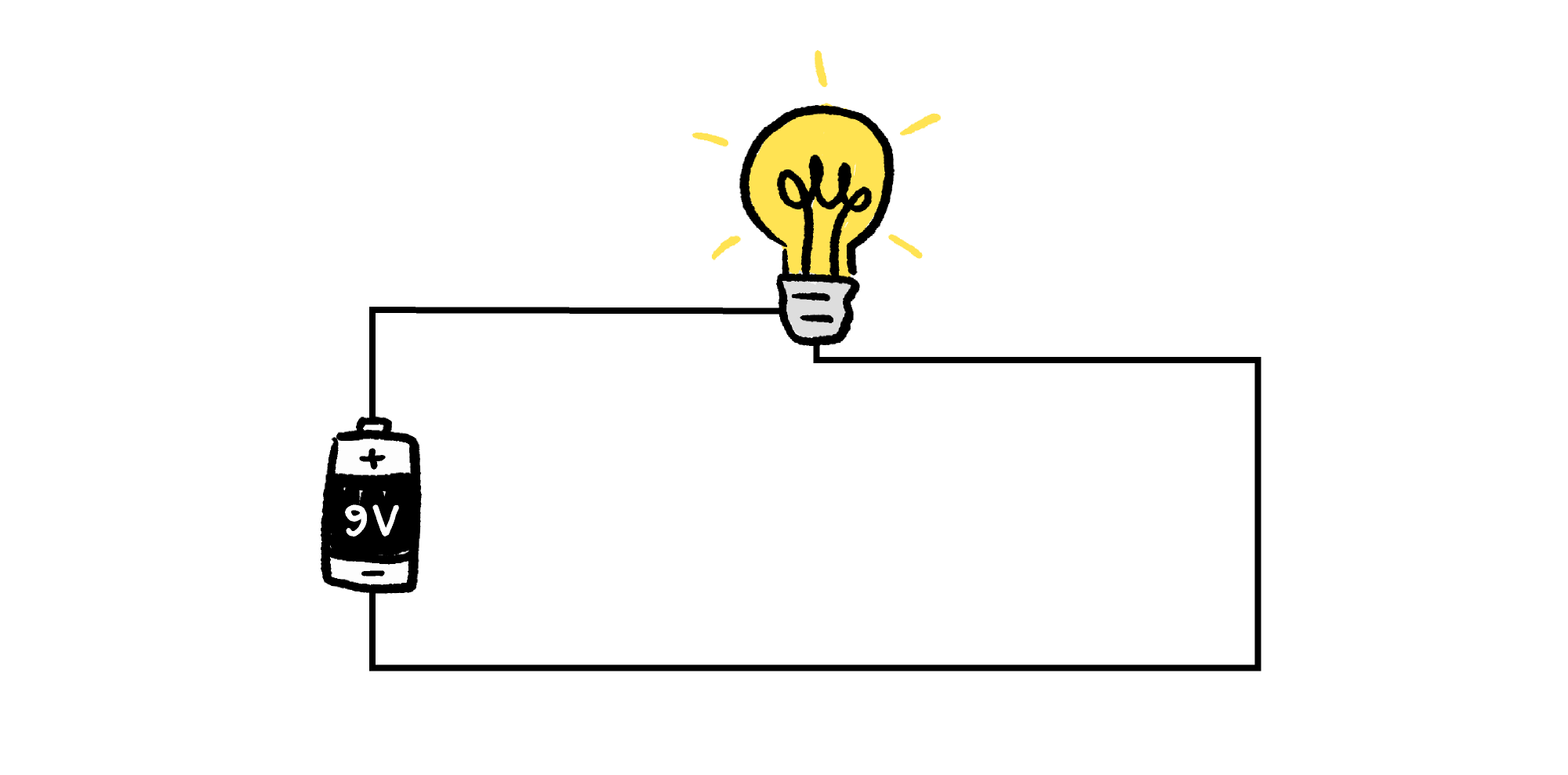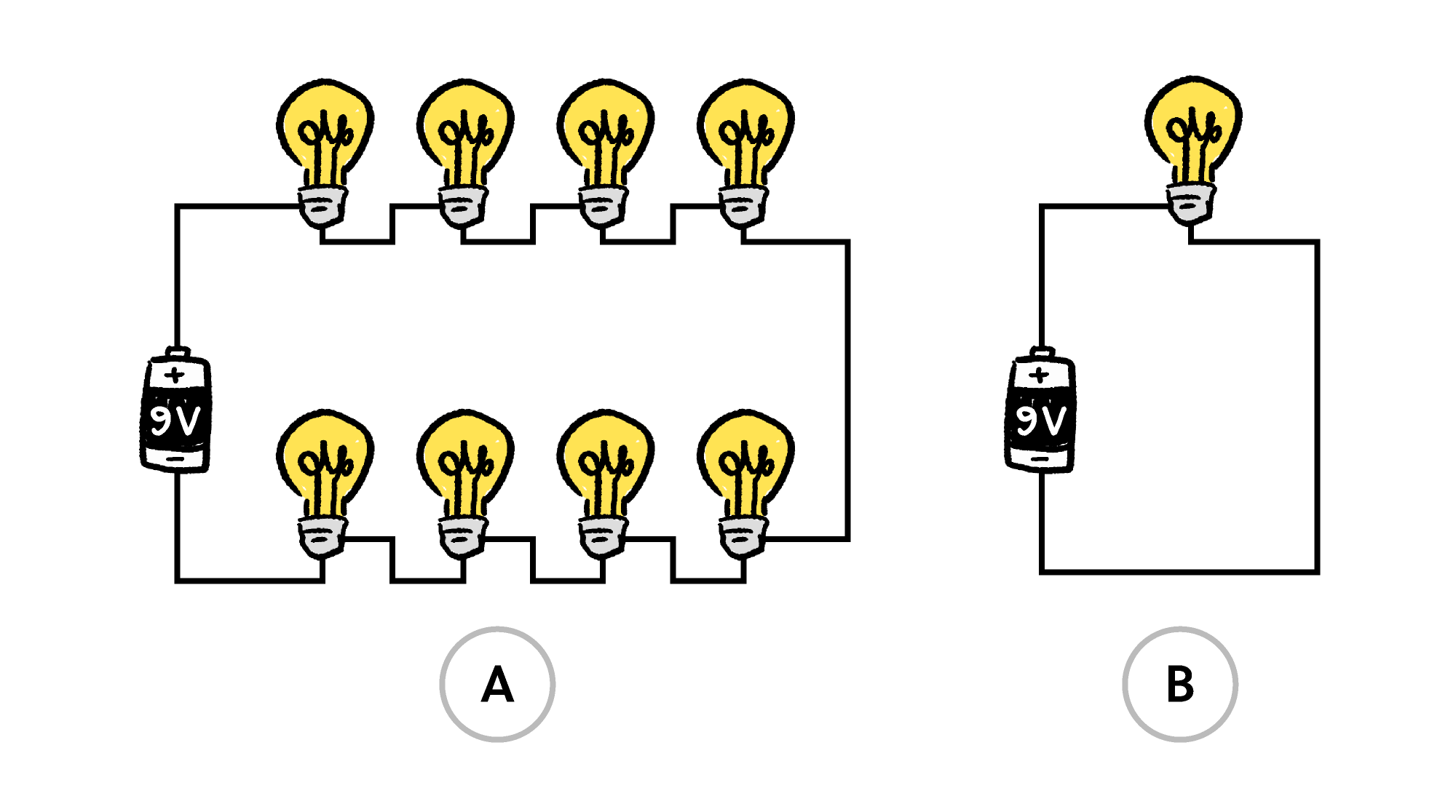Back

## Light Energy at Work

One of the first rules we learn in physics is that energy can never be created or destroyed, only transformed.

Much of the modern world is driven by transforming electricity into different forms of power. Speakers turn electrical power into sound. Light bulbs turn electrical power into light. Motors and generators turn electrical power into mechanical power and back, respectively. Keeping track of how electrical power is transferred and transformed is essential to making energy-efficient technologies.

A voltage source, like a battery or a generator, transports electrons through a wire in an electrical appliance. The rate that electric charge flows through a wire is called electric current $I.$Electrical power is produced as billions of individual electrons lose kinetic energy in collisions as they shuffle along. The rate that the electrons' energy is transformed — electrical power $P$ in a wire — is the product of the voltage $V$ across the wire and current $I:$ $P = IV.$

Light bulbs transform electrical power to light intensity, so the brightness of a bulb depends on the current and voltage in the circuit. A bulb's brightness is directly proportional to the power.The voltage of a $\SI{9}{\volt}$ battery forces electrons through a circuit, producing an electrical current. The light bulb resists the flow of electrons, decreasing the current that can flow through the circuit. All wires resist the flow of electrons, but not nearly so much as a light bulb.

The bulb’s resistance sets a limit on the current that can flow through the circuit with the battery’s voltage. If we put another identical bulb in series with the first, the resistance to the electrical current is doubled, and the maximum flow that can be sustained by the battery is cut in half.

If we add more identical bulbs, the current is reduced further, as the voltage is divided evenly among all the bulbs. The lights will illuminate, but at a lower intensity than a single bulb.

# Today's Challenge

Which arrangement uses the most electrical power and puts out the most light?We are sunsetting our community features by July 2, 2021. Learn more here.
×

Problem Loading...

Note Loading...

Set Loading...